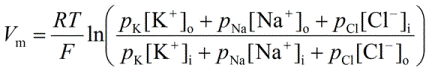PhysiologyWeb  Home  |  FAQ  |  About  |  Contact  |   - Sign InPhysiology Test Question
The following equation should be used to calculate the equilibrium potential for any given ion.
 (A)   The Nernst equation(B)   The Goldman-Hodgkin-Katz (GHK) equation